﻿ 基于软化桁架理论的人防框架梁受剪承载力分析

# 基于软化桁架理论的人防框架梁受剪承载力分析Shear Bearing Capacity Analysis of Protective Beams on the Basis of Softened Truss Theory

Abstract: On the basis of softened truss theory, established constitutive models for the softening charac-teristics of Fiber Reinforced Cementitious Composite (FRCC), High-stress Fine Grain Bar and FRCC Beams are studied with the application of strip method. Shear bearing capacity analysis is calculated with different sectional, FRCC strength, bar strength and reinforcement ratio. The re-sult manifested that: with the increase of the beam section, the strength of FRCC and the Bar, the shear bearing capacity increases linearly, but increasing slowly when the strength of the Bar exceeds 700 MPa; the compatibility of FRCC and the Bar influences entirety shear bearing capac-ity.

1. 引言

2. 基本理论和计算原理

2.1. 基本原理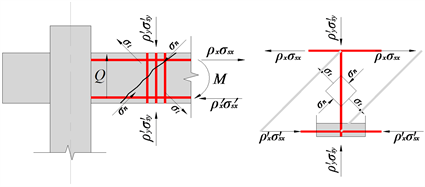Figure 1. Softened truss figure

2.2. 截面平衡方程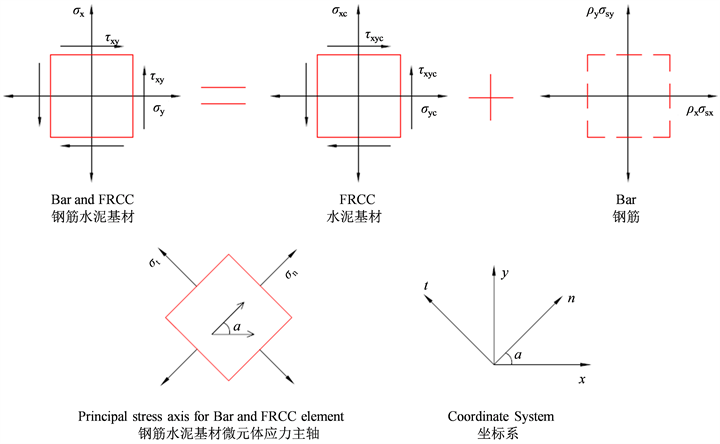Figure 2. Stress State of plane microelement for Bar and FRCC

$\left\{\begin{array}{l}{\sigma }_{x}={\sigma }_{t}{\mathrm{sin}}^{2}\alpha +{\sigma }_{n}{\mathrm{cos}}^{2}\alpha +{\rho }_{x}{\sigma }_{sx}\\ {\sigma }_{y}={\sigma }_{t}{\mathrm{cos}}^{2}\alpha +{\sigma }_{n}{\mathrm{sin}}^{2}\alpha +{\rho }_{y}{\sigma }_{sy}\\ {\tau }_{x}{}_{y}=\left({\sigma }_{n}-{\sigma }_{t}\right)\mathrm{sin}\alpha \cdot \mathrm{cos}\alpha \end{array}$ (1)

2.3. 变形协调方程

$\left\{\begin{array}{l}{\epsilon }_{x}={\epsilon }_{t}{\mathrm{sin}}^{2}\alpha +{\epsilon }_{n}{\mathrm{cos}}^{2}\alpha \\ {\epsilon }_{y}={\epsilon }_{t}{\mathrm{cos}}^{2}\alpha +{\epsilon }_{n}{\mathrm{sin}}^{2}\alpha \\ {\gamma }_{x}{}_{y}=\left({\epsilon }_{n}-{\epsilon }_{t}\right)\mathrm{sin}\alpha \cdot \mathrm{cos}\alpha \end{array}$ (2)

2.4. 材料本构关系

FRCC受压区应力—应变分段曲线为  ：

${\sigma }_{cf}=\left\{\begin{array}{l}{f}_{cf}\left[{\alpha }_{1}\xi +\left(3-2{\alpha }_{1}\right){\xi }^{2}+\left({\alpha }_{1}-2\right){\xi }^{3}\right]\text{ }\text{\hspace{0.17em}}\text{ }{\epsilon }_{cf}\le {\epsilon }_{0}{{}_{c}}_{f}\\ {f}_{cf}\frac{\xi }{{\alpha }_{2}{\left(\xi -1\right)}^{2}+\xi }\text{ }\text{ }\text{ }\text{ }\text{ }\text{ }\text{\hspace{0.17em}}\text{\hspace{0.17em}}\text{\hspace{0.17em}}\text{\hspace{0.17em}}\text{ }\text{\hspace{0.17em}}\text{\hspace{0.17em}}\text{\hspace{0.17em}}\text{\hspace{0.17em}}\text{\hspace{0.17em}}\text{\hspace{0.17em}}\text{\hspace{0.17em}}\text{\hspace{0.17em}}{\epsilon }_{c}{}_{f}>{\epsilon }_{0}{{}_{c}}_{f}\end{array}$ (3)

${\sigma }_{tf}=\left\{\begin{array}{l}{f}_{tf}\left({\alpha }_{0}+{\alpha }_{1}\xi +{\alpha }_{6}{\xi }^{6}\right)\text{ }\text{\hspace{0.17em}}\text{ }{\epsilon }_{tf}\le {\epsilon }_{0tf}\\ {f}_{tf}\frac{\xi }{{\alpha }_{t}{\left(\xi -1\right)}^{\beta }+\xi }\text{ }\text{ }\text{ }\text{\hspace{0.17em}}{\epsilon }_{tf}>{\epsilon }_{0tf}\end{array}$ (4)

${\sigma }_{n}=\left\{\begin{array}{l}\psi {f}_{cp}\left[{\alpha }_{1}\xi +\left(3-2{\alpha }_{1}\right){\xi }^{2}+\left({\alpha }_{1}-2\right){\xi }^{3}\right]\text{ }\text{ }\epsilon \le \psi {\epsilon }_{0}\\ \psi {f}_{cp}\frac{\xi }{{\alpha }_{2}{\left(\xi -1\right)}^{2}+\xi }\text{ }\text{ }\text{ }\text{ }\text{ }\text{ }\text{ }\text{ }\text{\hspace{0.17em}}\text{\hspace{0.17em}}\text{ }\text{\hspace{0.17em}}\text{ }\text{ }\text{ }\text{ }\epsilon >\psi {\epsilon }_{0}\end{array}$ (5)

2.5. 剪跨区内微元体的应力

${\tau }_{x}{}_{y}=\frac{Q}{b{h}_{0}}$ (6)

$a/h\le 0.5$ 时， ${k}_{1}=2dch$ ；当 $0.5 时， ${k}_{1}=\frac{dc}{h}\left(\frac{4h}{3a}-\frac{2}{3}\right)$ ；当 $a/h>2$ 时， ${k}_{1}=0$

${k}_{2}=\frac{dc}{2h}\left(1-0.3\lambda \frac{{h}_{0}}{h}\right)$ (7)

${\sigma }_{y}=k{\tau }_{xy}$ (8)

${\epsilon }_{x}=\frac{1}{2}\left({\epsilon }_{a}+{\epsilon }_{b}\right)$ (9)

${\sigma }_{x}=E{\epsilon }_{x}=\frac{E}{2}\left({\epsilon }_{a}+{\epsilon }_{b}\right)$ (10)

3. 软化桁架理论模型计算

$Q=\left\{\begin{array}{l}\left\{\psi {f}_{cp}\left[{\alpha }_{1}\xi +\left(3-2{\alpha }_{1}\right){\xi }^{2}+\left({\alpha }_{1}-2\right){\xi }^{3}\right]-{f}_{tp}\left({\alpha }_{0}+{\alpha }_{1}\xi +{\alpha }_{6}{\xi }^{6}\right)\right\}\mathrm{sin}\alpha \mathrm{cos}\alpha b{h}_{0}\text{ }\text{\hspace{0.17em}}\epsilon \le \psi {\epsilon }_{0}\\ \left(\psi {f}_{cp}\frac{\xi }{{\alpha }_{2}{\left(\xi -1\right)}^{2}+\xi }-{f}_{tp}\frac{\xi }{{\alpha }_{t}{\left(\xi -1\right)}^{\beta }+\xi }\right)\mathrm{sin}\alpha \mathrm{cos}\alpha b{h}_{0}\text{ }\text{ }\text{ }\text{ }\text{ }\text{ }\text{ }\text{ }\text{ }\text{\hspace{0.17em}}\epsilon >\psi {\epsilon }_{0}\end{array}$ (11)

① 每次取主压应力 ${\epsilon }_{n}:={\epsilon }_{n}+\Delta {\epsilon }_{n}$

② 假定εt值；

③ 根据 $\psi =0.9{\left(1+400{\epsilon }_{t}\right)}^{-0.5}$，求软化系数ψ；

④ 由本构关系求出σn、σt

⑤ 计算α；

⑥ 由σn、σt、α根据平衡方程解出τxy

⑦ 将每次计算的内力求和判断是否满足平衡条件；

⑧ 如不满足，修改假定的εt，重复③到⑦；

⑨ 由τxy，根据截面宽度及高度解出Q；

⑩ 重复①到⑨。

1) 人防框架梁的受剪承载力随着梁截面的增大基本呈线性增长，但随着剪跨比增大，其提高作用相对减小。

2) 人防框架梁的受剪承载力随着水泥基材强度的提高而增大，与fc或ft近似呈线性关系。

3) 和水泥基材强度的提高类似，人防框架梁的受剪承载力同样随着钢筋强度的提高而增大，但超过700 MPa后增长减缓，此时钢筋强度的计算值过高，和水泥基材强度已不匹配，影响到整个截面受剪承载力。

4) 箍筋对人防框架梁的受剪承载力有明显的提高作用，且两者具有线性关系，但剪跨比不同，配箍率对抗剪承载力的提高作用也稍有不同。

5) 在此次计算中，人防框架梁的受剪承载力随着纵筋配筋率的增大呈现出线性增长的趋势。由于剪切破坏的应力状态复杂，裂缝开展的多样性和不确定性，出于偏安全的考虑，现有设计方法一般不考虑纵筋对受剪承载力的影响，对于人防框架梁，纵向钢筋在构件受剪设计中的作用值得进一步研究。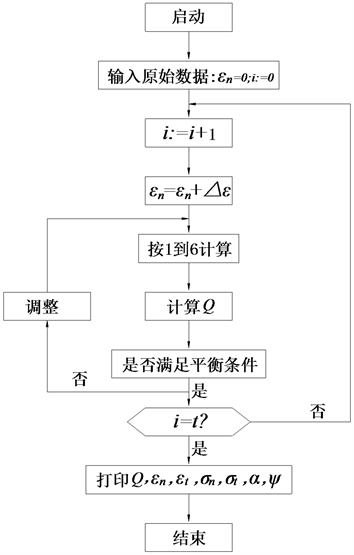Figure 3. Program block diagramTable 1. Shear bearing capacity with different sectional, FRCC strength, bar strength and reinforcement ratio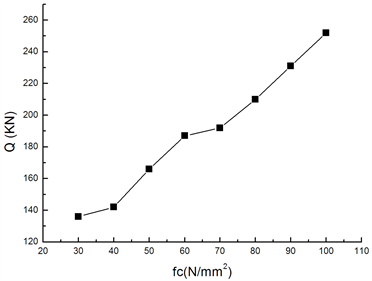(a)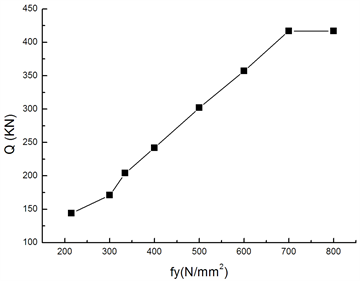(b)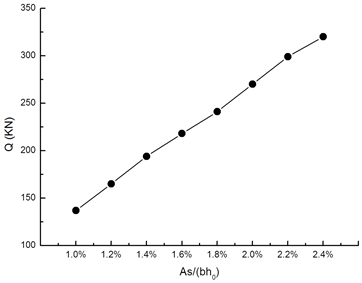(c)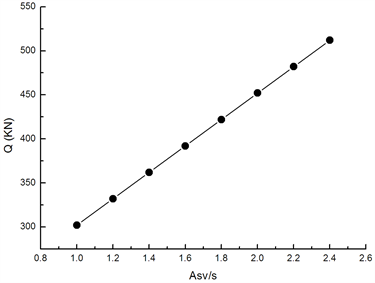(d)

Figure 4. Variety of shear capacity with different parameters. (a) Shear capacity with the strength of FRCC; (b) Shear capacity with the strength of Bar; (c) Shear capacity with the ratio of longitudinal bar; (d) Shear capacity with stirrup ratio

4. 结论

 Yao, S., Zhang, J.W. and Tu, Y.M. (2011) Discussion on HRBF500 Grain Reinforcement and C100 High-Performance Fiber Reinforced Cementitious Composites (HPFRCC) in the Civil Air Defense Work. Journal of Advanced Materials Research, 168-170, 1658-1664.
https://doi.org/10.4028/www.scientific.net/AMR.168-170.1658

 Thomas, T.C. (1988) Softened Truss Model Theory for Shear and Torsion. ACI Structural Journal, 85, 624-635.
https://doi.org/10.14359/2740

 姚山. 高强细晶粒钢筋与纤维增强水泥基复合材料在新型大跨度防护结构中的应用研究[D]: [博士学位论文]. 南京: 东南大学, 2013.

Top